• 图像旋转变换(matlab实现)

热门讨论 2012-03-27 14:31:02
使用matlab来实现一个图像旋转处理 一种几何变换 资源是matlab的一个m文件 代码都在里面 带有一点释放方便理解 :) 具体实现描述: http: blog csdn net abee23 article details 7398749 (支持原创哈) 测试平台...图像旋转 图像几何变换
• Matlab图像几何变换之图像旋转

万次阅读 多人点赞 2017-12-18 16:48:18
Matlab图像旋转 旋转原理如下： 点p0p_0绕坐标原点逆时针方向旋转θ\theta角度得到点p1p_1. 从①②可以得到旋转变换的矩阵公式： [x1y11]\begin{bmatrix}x_1&y_1&1\end{bmatrix}=[x0y01]\begin{b...

Matlab图像旋转
####以坐标原点为中心旋转的原理：
p 0 p_0 绕坐标原点逆时针方向旋转 θ \theta 角度得到点 p 1 p_1 .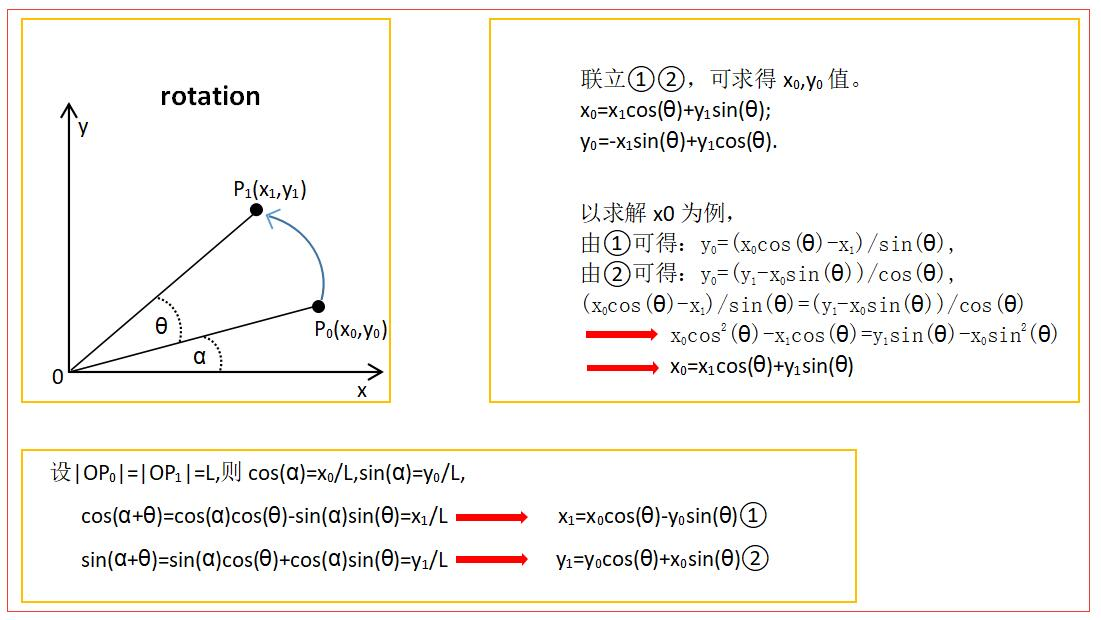从①②可以得到旋转变换的矩阵公式：
[ x 1 y 1 1 ] \begin{bmatrix}x_1&y_1&1\end{bmatrix} = [ x 0 y 0 1 ] \begin{bmatrix}x_0&y_0&1\end{bmatrix} [ c o s ( θ ) s i n ( θ ) 0 − s i n ( θ ) c o s ( θ ) 0 0 0 1 ] \begin{bmatrix}cos(\theta)&sin(\theta)&0\\-sin(\theta)&cos(\theta)&0\\0&0&1\end{bmatrix}

还可以得到逆运算矩阵公式：
[ x 0 y 0 1 ] \begin{bmatrix}x_0&y_0&1\end{bmatrix} = [ x 1 y 1 1 ] \begin{bmatrix}x_1&y_1&1\end{bmatrix} [ c o s ( θ ) − s i n ( θ ) 0 s i n ( θ ) c o s ( θ ) 0 0 0 1 ] \begin{bmatrix}cos(\theta)&-sin(\theta)&0\\sin(\theta)&cos(\theta)&0\\0&0&1\end{bmatrix}
####以任意图形中心点为坐标原点旋转原理：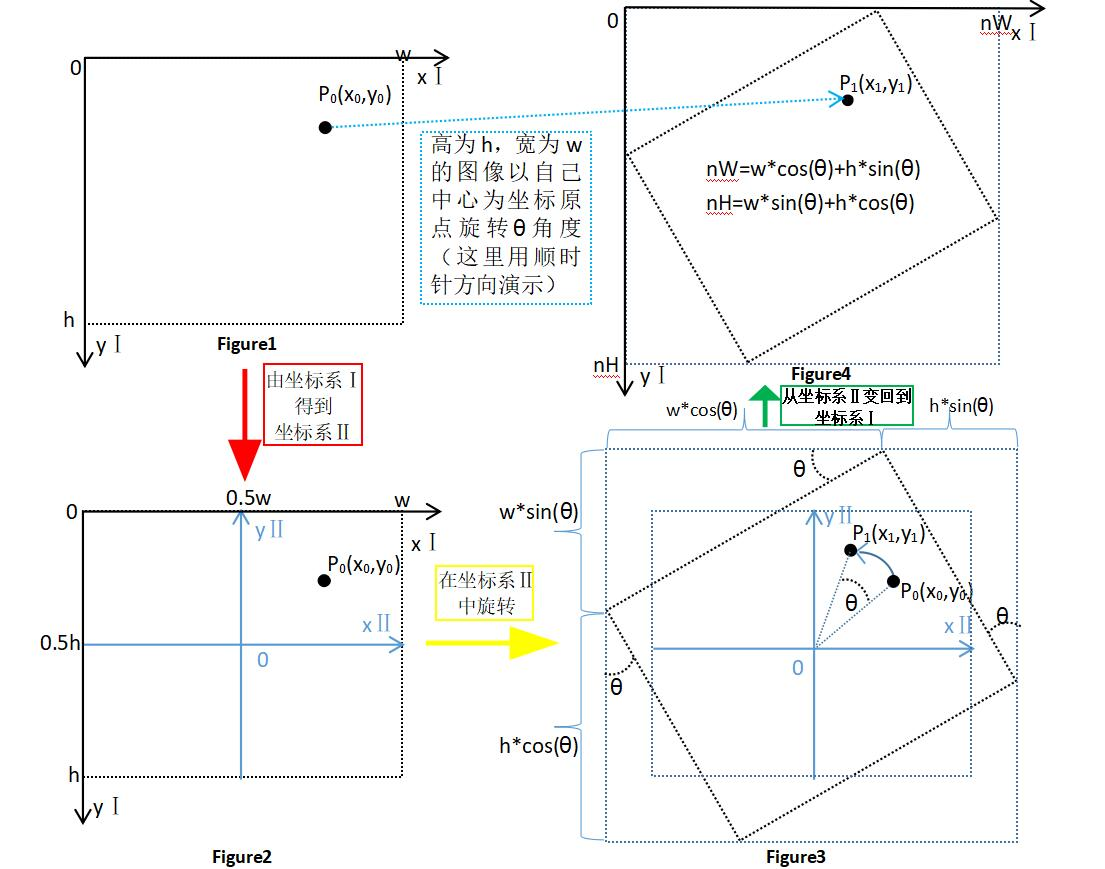从上图可知以任意图形中心点为坐标原点旋转我们需要三步：
(1)将坐标系Ⅰ变成坐标系Ⅱ
(2)在坐标系Ⅱ中旋转 θ \theta
(3)将坐标系Ⅱ变成坐标系Ⅰ

#####(1)将坐标系Ⅰ变成坐标系Ⅱ
由Figure1得到Figure2可知，变换矩阵为：
[ x Ⅱ y Ⅱ 1 ] \begin{bmatrix}xⅡ\\yⅡ\\1\end{bmatrix} = [ x Ⅰ y Ⅰ 1 ] \begin{bmatrix}xⅠ\\yⅠ\\1\end{bmatrix} [ 1 0 0 0 − 1 0 − 0.5 w 0.5 h 1 ] \begin{bmatrix}1&0&0\\0&-1&0\\-0.5w&0.5h&1\end{bmatrix} = [ x Ⅰ − 0.5 w − ( y Ⅰ − 0.5 h ) 1 ] \begin{bmatrix}xⅠ-0.5w\\-(yⅠ-0.5h)\\1\end{bmatrix}

#####(2)在坐标系Ⅱ中旋转 θ \theta
见上面以坐标原点为中心旋转的原理

#####(3)将坐标系Ⅱ变成坐标系Ⅰ
由Figure3得到Figure4可知，变换矩阵为(其实就是(1)中变换矩阵的逆变换)：
[ x Ⅰ y Ⅰ 1 ] \begin{bmatrix}xⅠ\\yⅠ\\1\end{bmatrix} = [ x Ⅱ y Ⅱ 1 ] \begin{bmatrix}xⅡ\\yⅡ\\1\end{bmatrix} [ 1 0 0 0 − 1 0 0.5 n W 0.5 n H 1 ] \begin{bmatrix}1&0&0\\0&-1&0\\0.5nW&0.5nH&1\end{bmatrix} = [ x Ⅱ + 0.5 n W − y Ⅱ + 0.5 n H 1 ] \begin{bmatrix}xⅡ+0.5nW\\-yⅡ+0.5nH\\1\end{bmatrix}

从而将三步合起来，可以得到以任意图像中心为坐标原点旋转的变换矩阵：
[ x 1 y 1 1 ] \begin{bmatrix}x_1\\y_1\\1\end{bmatrix} = [ x 0 y 0 1 ] \begin{bmatrix}x_0\\y_0\\1\end{bmatrix} [ 1 0 0 0 − 1 0 − 0.5 w 0.5 h 1 ] \begin{bmatrix}1&0&0\\0&-1&0\\-0.5w&0.5h&1\end{bmatrix} [ c o s ( θ ) s i n ( θ ) 0 − s i n ( θ ) c o s ( θ ) 0 0 0 1 ] \begin{bmatrix}cos(\theta)&sin(\theta)&0\\-sin(\theta)&cos(\theta)&0\\0&0&1\end{bmatrix}
[ 1 0 0 0 − 1 0 0.5 n W 0.5 n H 1 ] \begin{bmatrix}1&0&0\\0&-1&0\\0.5nW&0.5nH&1\end{bmatrix} = [ x 0 y 0 1 ] \begin{bmatrix}x_0\\y_0\\1\end{bmatrix} [ c o s ( θ ) − s i n ( θ ) 0 s i n ( θ ) c o s ( θ ) 0 − 0.5 w c o s ( θ ) − 0.5 h s i n ( θ ) + 0.5 n W − 0.5 w s i n ( θ ) + 0.5 h c o s ( θ ) + 0.5 n H 1 ] \begin{bmatrix}cos(\theta)&-sin(\theta)&0\\sin(\theta)&cos(\theta)&0\\-0.5wcos(\theta)-0.5hsin(\theta)+0.5nW&-0.5wsin(\theta)+0.5hcos(\theta)+0.5nH&1\end{bmatrix}

它的逆变换为：
[ x 0 y 0 1 ] \begin{bmatrix}x_0\\y_0\\1\end{bmatrix} = [ x 1 y 1 1 ] \begin{bmatrix}x_1\\y_1\\1\end{bmatrix} [ 1 0 0 0 − 1 0 − 0.5 n W 0.5 n H 1 ] \begin{bmatrix}1&0&0\\0&-1&0\\-0.5nW&0.5nH&1\end{bmatrix} [ c o s ( θ ) − s i n ( θ ) 0 s i n ( θ ) c o s ( θ ) 0 0 0 1 ] \begin{bmatrix}cos(\theta)&-sin(\theta)&0\\sin(\theta)&cos(\theta)&0\\0&0&1\end{bmatrix}
[ 1 0 0 0 − 1 0 0.5 w 0.5 h 1 ] \begin{bmatrix}1&0&0\\0&-1&0\\0.5w&0.5h&1\end{bmatrix} = [ x 0 y 0 1 ] \begin{bmatrix}x_0\\y_0\\1\end{bmatrix} [ c o s ( θ ) s i n ( θ ) 0 − s i n ( θ ) c o s ( θ ) 0 − 0.5 n W c o s ( θ ) + 0.5 n H s i n ( θ ) + 0.5 w − 0.5 n W s i n ( θ ) − 0.5 n H c o s ( θ ) + 0.5 h 1 ] \begin{bmatrix}cos(\theta)&sin(\theta)&0\\-sin(\theta)&cos(\theta)&0\\-0.5nWcos(\theta)+0.5nHsin(\theta)+0.5w&-0.5nWsin(\theta)-0.5nHcos(\theta)+0.5h&1\end{bmatrix}

####Matlab编程实现
#####(1)Matlab自带函数实现图像任意角度旋转
######旋转函数介绍：
B=imrotate(A,angle,method, ‘crop’)
angle   :旋转角度，单位为度，角度为正值时逆时针旋转
method :该可选参数为imrotate()指定插值方法
‘crop’:裁剪旋转后增大的图像

clc
figure,imshow(I);
title('srcImage');
I1=imrotate(I,30);                  %旋转30°
I2=imrotate(I,30,'crop');           %旋转30°，并剪切图像，使得到的图像和原图像大小一致
I3=imrotate(I,30,'bilinear','crop');%双线性插值法旋转30°，并剪切图像，使得到的图像和原图像大小一致
figure,imshow(I1);
title('I1');
figure,imshow(I2);
title('I2');
figure,imshow(I3);
title('I3');

程序运行结果如下：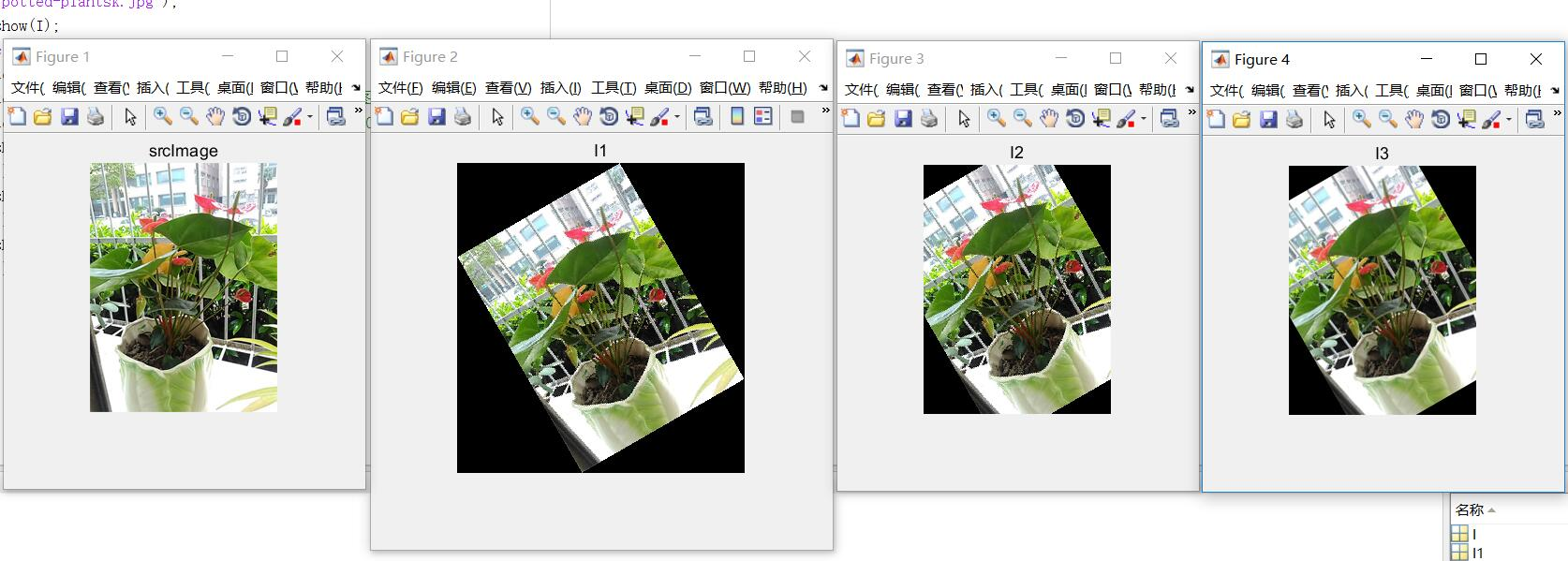#####(2)自编myimrotate()函数实现图像任意角度旋转

function [ A ] = myimrotate(B,degree)                                 %定义旋转函数，degree为要旋转的角度
[r,c,d]=size(B);                                                      %获取输入图像B的行r、列c和通道数d,为了旋转彩色图像所以有必要得到通道数d
nH=round(r*abs(cosd(degree))+c*abs(sind(degree)));                    %旋转图像后得到的新高度，“round()函数四舍五入“
nW=round(c*abs(cosd(degree))+r*abs(sind(degree)));                    %旋转图像后得到的新宽度
A=zeros(nH,nW,d);                                                     %定义生成目标图像的行列以及通道数
M1=[1 0 0;0 -1 0;-0.5*nW 0.5*nH 1 ];                                  %坐标系变换矩阵M1
M2=[cosd(degree) -sind(degree) 0;sind(degree) cosd(degree) 0;0 0 1];  %角度旋转变换矩阵M2，我用的是顺时针方向
M3=[1 0 0;0 -1 0;0.5*c 0.5*r 1];                                      %坐标系变换矩阵M3
for i=1:nW
for j=1:nH
temp=[i j 1]*M1*M2*M3;                                    %得到旋转后的矩阵temp
y=temp(1,2);                                              %y取矩阵temp的第一行第二列,y对应j，为高度
x=temp(1,1);                                              %x取矩阵temp的第一行第一列,x对应i，为宽度
y=round(y);                                               %y四舍五入取整
x=round(x);                                               %x四舍五入取整
if(x>=1&&x<=c)&&(y>=1&&y<=r)                               %判断的得到的(x,y)点是否在原图像上
A(j,i,:)=B(y,x,:);                                     %将原图像的像素点赋值给对应的旋转后图像上的点
end                                                        %（”有人疑惑为啥不是A(i,j,:)=B(x,y,:);因为i,x对应的是列，即宽，而j,y对应的是行，即高“），我这里以x为横坐标，y为竖向纵坐标
end
end
end

调用函数：

clc
figure,imshow(I);
title('srcImage');
I1=myimrotate(I,30);     %调用myimrotate()函数旋转30°
I2=myimrotate(I,-90);     %调用myimrotate()函数旋转-90°
figure,imshow(uint8(I1));
title('旋转30°：I1');
figure,imshow(uint8(I2));
title('旋转-90°：I2');

程序运行结果：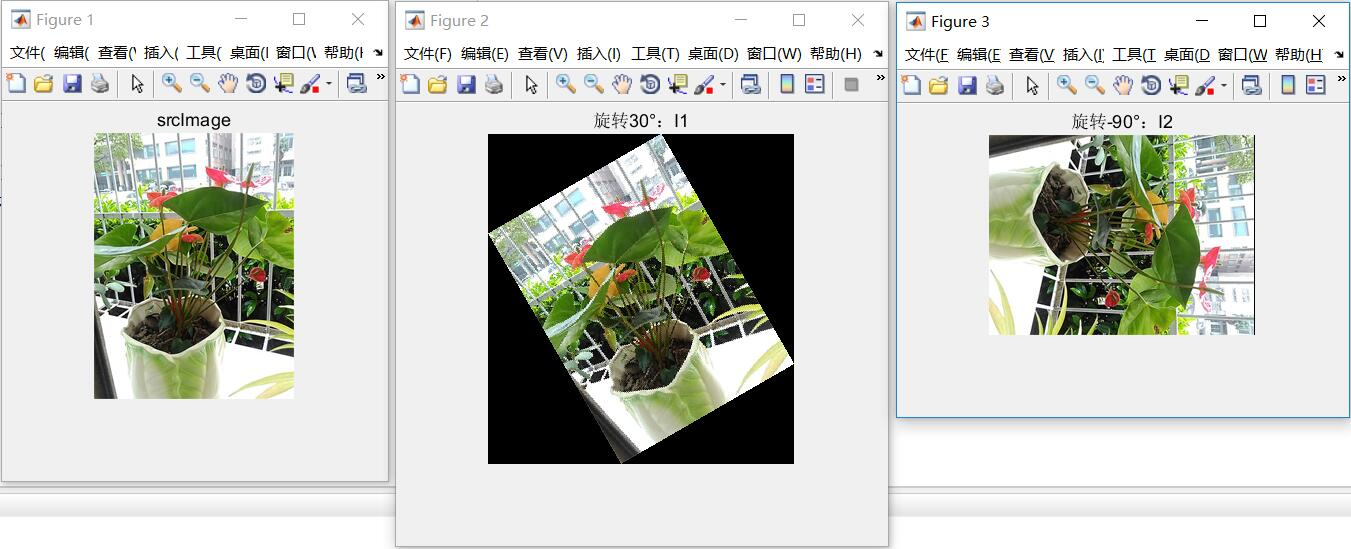补充一下：
sin/cos/tan                     三角函数，角度单位为弧度
asin/acos/atan                反三角函数，角度单位为弧度

sind/cosd/tand               三角函数，角度单位为度
asind/acosd/atand          反三角函数，角度单位为度

补充：坐标系平移推导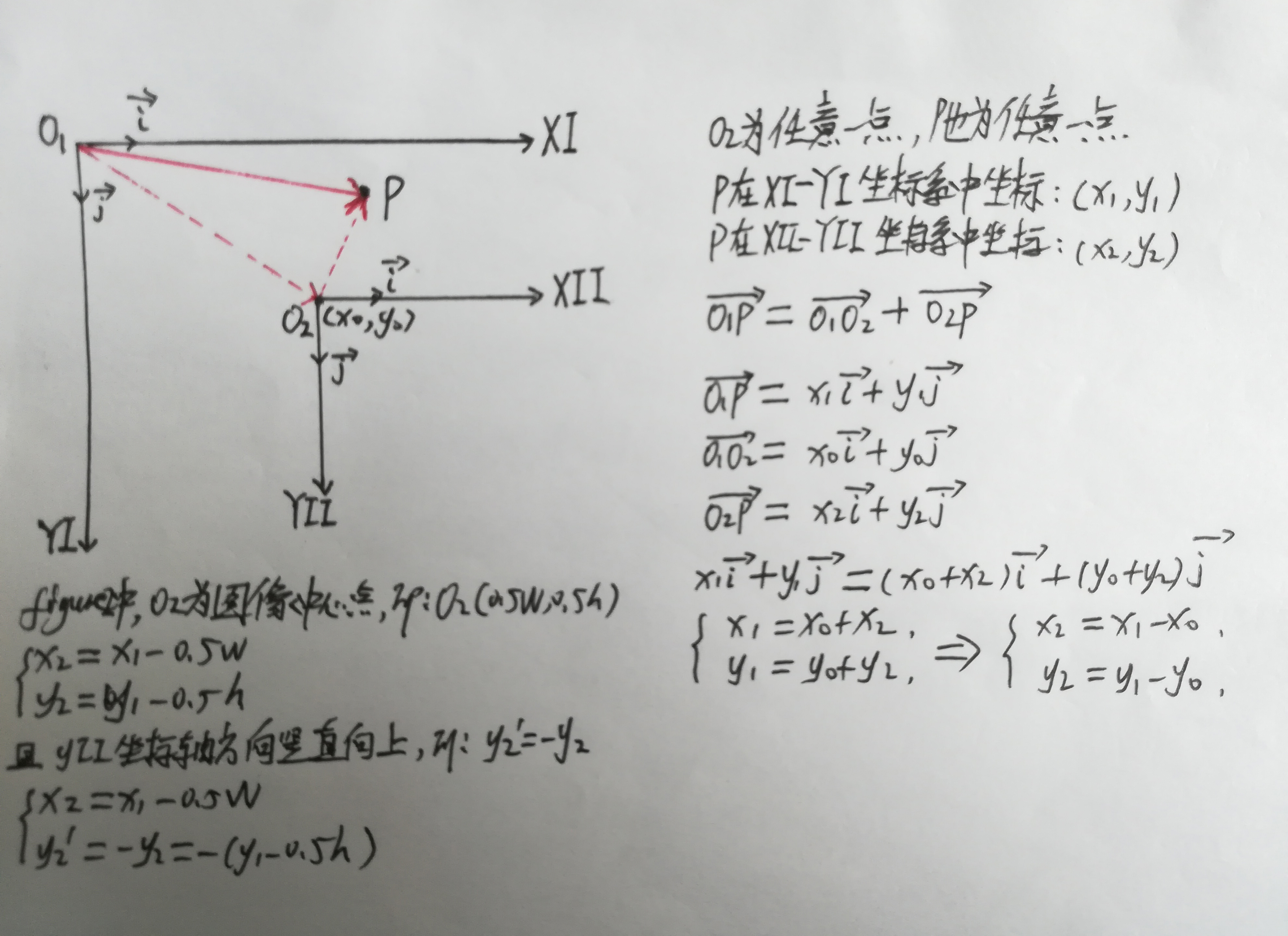展开全文matlab
• 图像旋转原理及实现

万次阅读 多人点赞 2016-01-21 17:57:14
在一次数字图像处理课中，我接触到了图片旋转的一些原理，当时没完全想明白，课后通过一段时间的学习，终于完成了图片旋转matlab程序。开始觉得应该挺简单的，但终究是纸上谈兵，在实现的过程中遇到很多问题。

建议阅读该博客的朋友最好对插值、matlab编程、数字图像有一些了解，另外所有代码和测试图片都可以到GitHub去下载。

在一次数字图像处理课中，我接触到了图片旋转的一些原理，当时没完全想明白，课后通过一段时间的学习，终于完成了图片旋转的matlab程序。开始觉得应该挺简单的，但终究是纸上谈兵，在实现的过程中遇到很多问题。

1 旋转矩形

首先建议阅读 图像旋转算法原理-旋转矩阵，这篇博客可以让你很好地理解图像中的每一个点是如何进行旋转操作的。其中涉及到了图像原点与笛卡尔坐标原点之间的相互转换以及点旋转的一些公式推导。

我们一般认为图像应该绕着中心点旋转，但是图像的原点在左上角，在计算的时候首先需要将左上角的原点移到图像中心，并且Y轴需要翻转。设一点 ( X 0 , Y 0 ) (X_0,Y_0) ，图像宽为 W W ，高为 H H ，原点变换后的点为 ( X 1 , Y 1 ) (X_1,Y_1) ，变换如下式。

(1) [ X 1 Y 1 1 ] = [ X 0 Y 0 1 ] [ 1 0 0 0 − 1 0 − 0.5 W 0.5 H 1 ] \begin{bmatrix} X_1 &amp; Y_1 &amp; 1 \end{bmatrix} = \begin{bmatrix} X_0 &amp; Y_0 &amp; 1 \end{bmatrix} \begin{bmatrix} 1 &amp; 0 &amp; 0 \\ 0 &amp; -1 &amp; 0 \\ -0.5W &amp; 0.5H &amp; 1 \end{bmatrix} \tag{1}

图像旋转角度为 θ \theta ，设原点变换后通过旋转矩阵旋转 θ \theta 后的点为 ( X 2 , Y 2 ) (X_2,Y_2) ，公式如下。

(2) [ X 2 Y 2 1 ] = [ X 1 Y 1 1 ] [ c o s θ − s i n θ 0 s i n θ c o s θ 0 0 0 1 ] \begin{bmatrix} X_2 &amp; Y_2 &amp; 1 \end{bmatrix} = \begin{bmatrix} X_1 &amp; Y_1 &amp; 1 \end{bmatrix} \begin{bmatrix} cos\theta &amp; -sin \theta &amp; 0 \\ sin\theta &amp; cos\theta &amp; 0 \\ 0 &amp; 0 &amp; 1 \end{bmatrix} \tag{2}

旋转后的图像的宽为 W &quot; W^&quot; ，高为 H &quot; H^&quot; ，那么从笛卡尔坐标原点变换回左上角的公式如下。

(3) [ X 3 Y 3 1 ] = [ X 2 Y 2 1 ] [ 1 0 0 0 − 1 0 0.5 W &quot; 0.5 H &quot; 1 ] \begin{bmatrix} X_3 &amp; Y_3 &amp; 1 \end{bmatrix} = \begin{bmatrix} X_2 &amp; Y_2 &amp; 1 \end{bmatrix} \begin{bmatrix} 1 &amp; 0 &amp; 0 \\ 0 &amp; -1 &amp; 0 \\ 0.5W^&quot; &amp; 0.5H^&quot; &amp; 1 \end{bmatrix} \tag{3}

综上可得，原图像的一点 ( X 0 , Y 0 ) (X_0,Y_0) 经过如下公式可以变换到旋转后的 ( X 3 , Y 3 ) (X_3,Y_3)

(4) [ X 3 Y 3 1 ] = [ X 0 Y 0 1 ] ∗ [ 1 0 0 0 − 1 0 − 0.5 W 0.5 H 1 ] [ c o s θ − s i n θ 0 s i n θ c o s θ 0 0 0 1 ] [ 1 0 0 0 − 1 0 0.5 W &quot; 0.5 H &quot; 1 ] \begin{bmatrix} X_3 &amp; Y_3 &amp; 1 \end{bmatrix} = \begin{bmatrix} X_0 &amp; Y_0 &amp; 1 \end{bmatrix} * \begin{bmatrix} 1 &amp; 0 &amp; 0 \\ 0 &amp; -1 &amp; 0 \\ -0.5W &amp; 0.5H &amp; 1 \end{bmatrix} \begin{bmatrix} cos\theta &amp; -sin \theta &amp; 0 \\ sin\theta &amp; cos\theta &amp; 0 \\ 0 &amp; 0 &amp; 1 \end{bmatrix} \begin{bmatrix} 1 &amp; 0 &amp; 0 \\ 0 &amp; -1 &amp; 0 \\ 0.5W^&quot; &amp; 0.5H^&quot; &amp; 1 \end{bmatrix} \tag{4}

同理，旋转后的一点 ( X 3 , Y 3 ) (X_3,Y_3) 经过如下公式可以变换回原图像的 ( X 0 , Y 0 ) (X_0,Y_0)

(5) [ X 0 Y 0 1 ] = [ X 3 Y 3 1 ] ∗ [ 1 0 0 0 − 1 0 − 0.5 W &quot; 0.5 H &quot; 1 ] [ c o s θ s i n θ 0 − s i n θ c o s θ 0 0 0 1 ] [ 1 0 0 0 − 1 0 0.5 W 0.5 H 1 ] \begin{bmatrix} X_0 &amp; Y_0 &amp; 1 \end{bmatrix} = \begin{bmatrix} X_3 &amp; Y_3 &amp; 1 \end{bmatrix} * \begin{bmatrix} 1 &amp; 0 &amp; 0 \\ 0 &amp; -1 &amp; 0 \\ -0.5W^&quot; &amp; 0.5H^&quot; &amp; 1 \end{bmatrix} \begin{bmatrix} cos\theta &amp; sin \theta &amp; 0 \\ -sin\theta &amp; cos\theta &amp; 0 \\ 0 &amp; 0 &amp; 1 \end{bmatrix} \begin{bmatrix} 1 &amp; 0 &amp; 0 \\ 0 &amp; -1 &amp; 0 \\ 0.5W &amp; 0.5H &amp; 1 \end{bmatrix} \tag{5}

在开始下一部分之前，先要得到旋转后图像的大小，设原图像高为m，宽为n。代码如下，虽然看起来有点复杂，建议朋友们自己画张图看一下，那样会一目了然。

% image size after rotation
[m, n, o] = size(img);
new_m = ceil(abs(m*cosd(degree)) + abs(n*sind(degree)));
new_n = ceil(abs(n*cosd(degree)) + abs(m*sind(degree)));

2 前向映射

建议阅读 图像旋转变换-matlab实现，里面采用的是前向映射的方法。

什么是前向映射呢？就是通过原图像的坐标计算旋转之后的坐标，并将相应的灰度值传给旋转后的图像。这样遇到最大的问题就是出现了一些有规律的噪声，如下图。通过代码来分析一下产生这种现象的原因，前向映射的代码如下。m1，m2和m3这三个矩阵可以对应到公式(4)中的三个矩阵，可以看出转换后的坐标是小数，并且进行了取整操作，这样部分像素并没有对应的灰度值。

% forward mapping matrices
m1 = [1 0 0; 0 -1 0; -0.5*n 0.5*m 1];
m2 = [cosd(degree) -sind(degree) 0; sind(degree) cosd(degree) 0; 0 0 1];
m3 = [1 0 0; 0 -1 0; 0.5*new_n 0.5*new_m 1];

for i = 1:n
for j = 1:m
new_coordinate = [i j 1]*m1*m2*m3;
col = round(new_coordinate(1));
row = round(new_coordinate(2));
% no-rotation image's coordinates to rotated image's coordinates
new_img_forward(row, col, 1) = img(j, i, 1);
new_img_forward(row, col, 2) = img(j, i, 2);
new_img_forward(row, col, 3) = img(j, i, 3);
end
end

3 反向映射

为了解决上述问题，可以采用反向映射的方法，即从旋转后的图像出发，找到对应的原图像的点，然后将原图像中的灰度值传递过来即可，这样旋转后的图像的每个像素肯定可以对应到原图像中的一个点，采取不同策略可以让像素对应地更加准确，建议首先阅读 图像旋转算法与实现以及 基于Matlab的双线性插值算法在图像旋转中的应用来更好的理解反向映射。

下图可以很好地理解反向映射的过程。下面代码实现了最近邻插值和双线性插值，其中rm1，rm2和rm3表示式(5)中的三个转换矩阵。

% reverse mapping matrices
rm1 = [1 0 0; 0 -1 0; -0.5*new_n 0.5*new_m 1];
rm2 = [cosd(degree) sind(degree) 0; -sind(degree) cosd(degree) 0; 0 0 1];
rm3 = [1 0 0; 0 -1 0; 0.5*n 0.5*m 1];

for i = 1:new_n
for j = 1:new_m
% rotated image's coordinates to no-rotation image's coordinates
old_coordinate = [i j 1]*rm1*rm2*rm3;
col = round(old_coordinate(1));
row = round(old_coordinate(2));
% prevent border overflow
if row < 1 || col < 1 || row > m || col > n
new_img_nnp(j, i) = 0;
new_img_lp(j, i) = 0;
else
% nearest neighbor interpolation
new_img_nnp(j, i, 1) = img(row, col, 1);
new_img_nnp(j, i, 2) = img(row, col, 2);
new_img_nnp(j, i, 3) = img(row, col, 3);

% bilinear interpolation
left = floor(col);
right = ceil(col);
top = floor(row);
bottom = ceil(row);

a = col - left;
b = row - top;
new_img_lp(j, i, 1) = (1-a)*(1-b)*img(top, left, 1) + a*(1-b)*img(top, right, 1) + ...
(1-a)*b*img(bottom, left, 1) + a*b*img(bottom, right, 1);
new_img_lp(j, i, 2) = (1-a)*(1-b)*img(top, left, 2) + a*(1-b)*img(top, right, 2) + ...
(1-a)*b*img(bottom, left, 2) + a*b*img(bottom, right, 2);
new_img_lp(j, i, 3) = (1-a)*(1-b)*img(top, left, 3) + a*(1-b)*img(top, right, 3) + ...
(1-a)*b*img(bottom, left, 2) + a*b*img(bottom, right, 3);
end
end
end

经过反向映射得到如下结果，下面展示的是反向映射+双线性插值的结果，很好的解决了前向映射出现有规律噪声的问题。4 总结

文章介绍了关于图像旋转的一些原理和相关的实现，从旋转矩阵开始，到前向映射，再到反向映射，反向映射中采用了最近邻插值和双线性插值，较好的实现了图片的旋转功能。建议朋友们多阅读文中引用的一些博客和论文，这样对图像旋转的很多细节会有更好的把握。
展开全文图像旋转 矩阵旋转 matlab 数字图像
• MATLAB实现图像旋转

千次阅读 多人点赞 2020-01-23 22:15:41
一、图像旋转原理 图像旋转的本质是向量的旋转。直接看图 向量的旋转很容易理解，在图像旋转中，图像中心到某一像素点就可视作一向量，对该向量进行关于中点的旋转即可对该像素点进行旋转，对所有像素点进行旋转...

一、图像旋转的原理

图像旋转的本质是向量的旋转。直接看图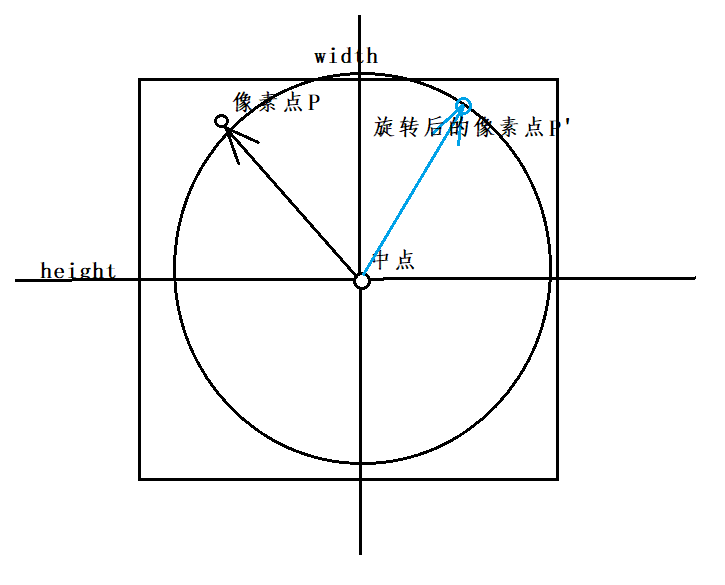向量的旋转很容易理解，在图像旋转中，图像中心到某一像素点就可视作一向量，对该向量进行关于中点的旋转即可对该像素点进行旋转，对所有像素点进行旋转就完成了图像的旋转。

方案一、正向直接旋转

设原像素点P对应向量为K1,旋转后像素点P’对应向量为K2,旋转矩阵R为：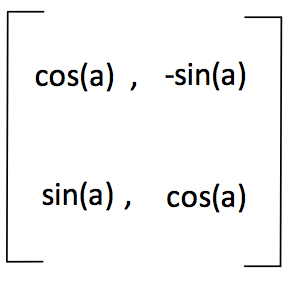则:可以得到 K 2 = R ∗ K 1 K2=R*K1
看下图，若相对整张图片P位置为p1,P’位置为p2,图像中点位置为c,则可以得到：
p 2 − c = R ( p 1 − c ) p2-c=R(p1-c)
得到： p 2 = R ( p 1 − c ) + c p2=R(p1-c)+c
正向法的思路是将原图片中的每一个像素点做旋转变换求出其在新图片中的位置，再赋给新图片的对应位置。
中点坐标可以由图片宽度 w i d t h width 和高度 h e i g h t height 得到。
MATLAB代码如下：

%读入图片

%旋转30°
%求出旋转矩阵
a=30/180*pi;
R=[cos(a),-sin(a);sin(a),cos(a)];%旋转矩阵

%求出图片大小
sz=size(im);
height=sz(1);%高度
width=sz(2);%宽度
channel=sz(3);%通道数

center=[height;width]/2;%求出图片中心位置

%构造旋转结果图像
im2=uint8(zeros(height,width,3));
for k = 1:channel
for i = 1:height
for j = 1:width
p=[i;j];%遍历每一个像素点
%round为四舍五入
pp=round(R*(p-center)+center);%旋转，求出结果坐标
%这里简单用了round函数来取整，因此旋转结果非常不理想
%排除不在画布内的像素
if(pp(1)>=1 && pp(1)<=height &&pp(2)>=1 && pp(2) <= width)
im2(pp(1),pp(2),k)=im(i,j,k);
end
end
end
end

figure;
imshow(im);
figure;
imshow(im2);

结果如下：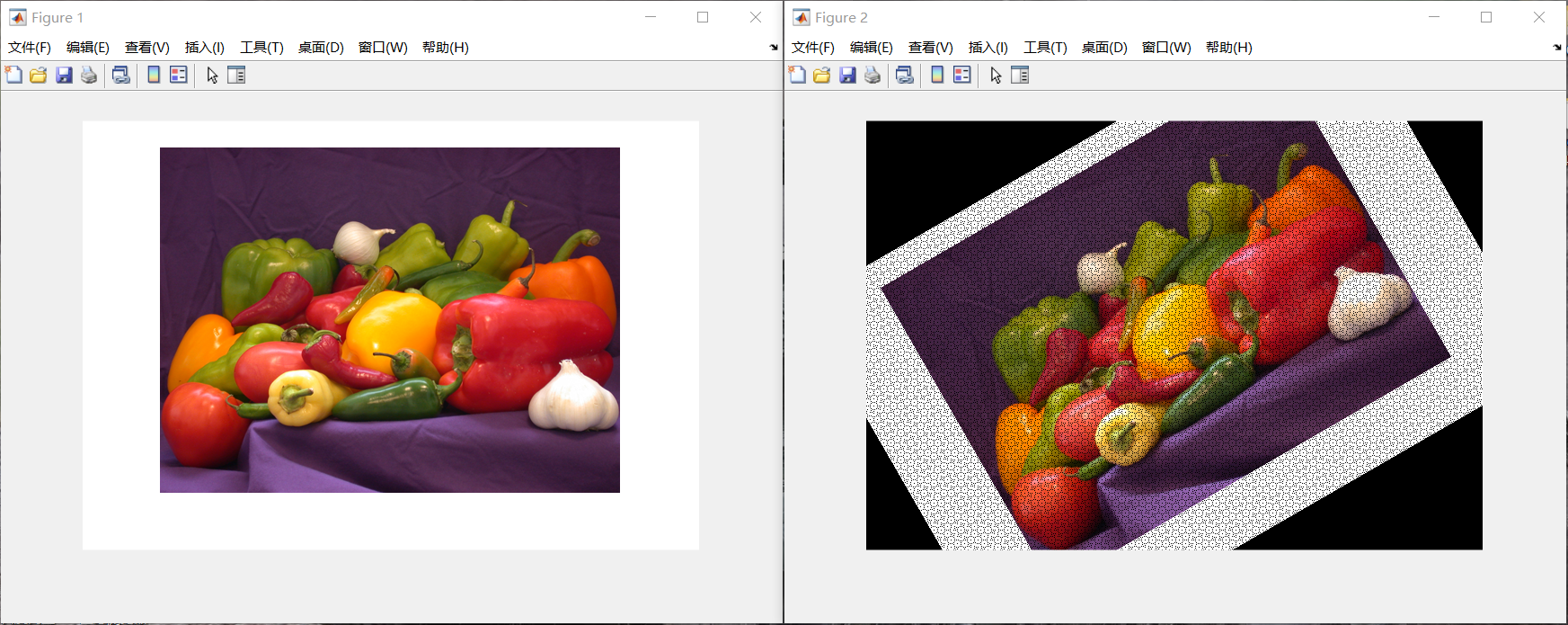可以看出，按照方案一旋转后的图片有几个问题：
1、图片旋转后由一部分被切割了，无法正常显示
2、新图片某些像素无法显示
具体见下图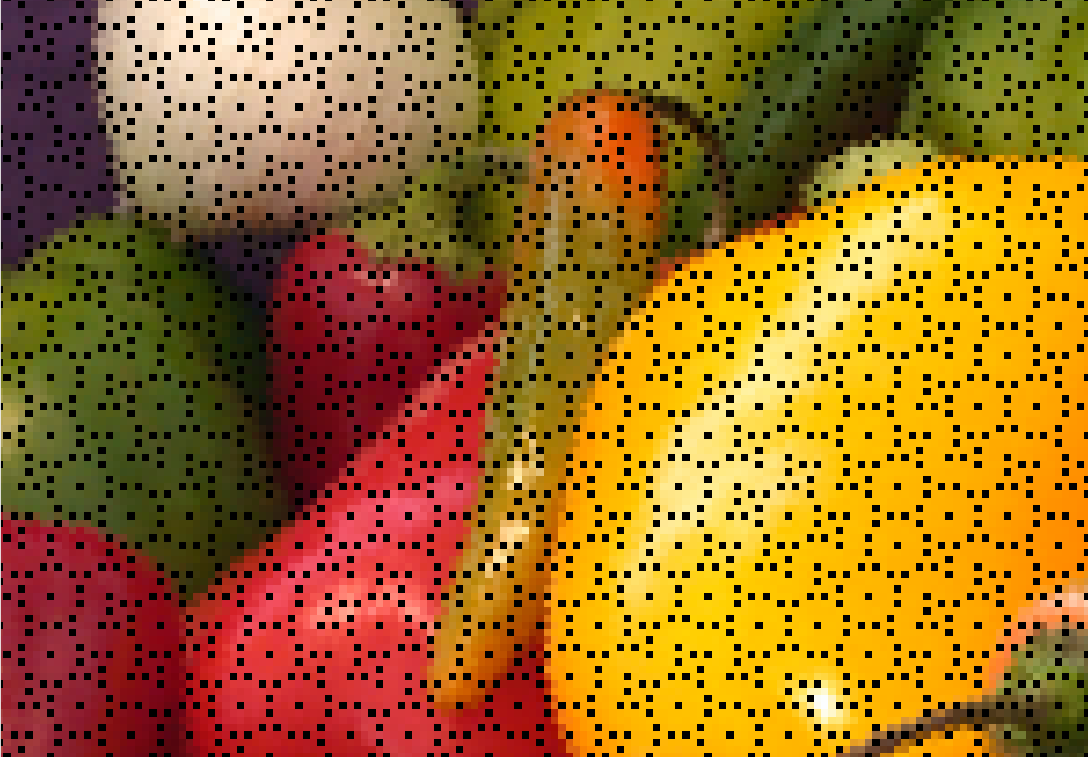导致新图像显示不完全的原因是图像在旋转后需要的画布大小发生了变化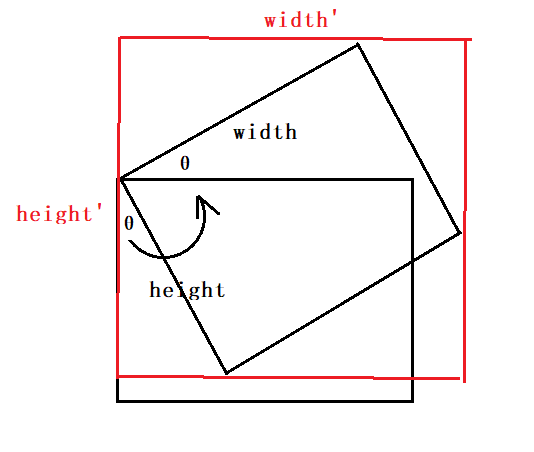由上面这张图很容易可以得出：
w i d t h ′ = w i d t h ∗ c o s θ + h e i g h t ∗ s i n θ ; width'=width*cosθ+height*sinθ;
h e i g h t ′ = h e i g h t ∗ c o s θ + w i d t h ∗ s i n θ ; height'=height*cosθ+width*sinθ;

而新图像部分像素点无法显示的原因是在求旋转后像素点坐标时使用round函数粗略地求出新坐标的整数值，而导致部分原像素计算后的新像素位置重叠，进而导致新图片中部分点像素缺失。并且从结果来看缺失像素点非常多。
解决这个问题有两种新方案：

方案二、反向查找法

与方案一正向法不同的是，方案二的思路正好相反，求出新图像每一个像素点旋转前在原图片中的位置，再将原图片中对应像素赋值给新图片
并且需要重新计算新画布的大小和新画布的中点位置c2

若旋转矩阵的逆矩阵为R’，则可以得出
K 1 = R ′ ∗ K 2 ; K1=R'*K2;
换算成位置就是 p 1 − c 1 = R ∗ ( p 2 − c 2 ) p1-c1=R*(p2-c2)
得出： p 1 = R ∗ ( p 2 − c 2 ) + c 1 ; p1=R*(p2-c2)+c1;
MATLAB代码：

%旋转30°
%求出旋转矩阵
a=30/180*pi;
R=[cos(a),-sin(a);sin(a),cos(a)];%旋转矩阵
R=R';%求出旋转矩阵的逆矩阵

%求出图片大小
sz=size(im);
h=sz(1);
w=sz(2);
ch=sz(3);
c1=[h;w]/2;%原图中心

%计算显示完整图像需要的画布大小
hh=floor(width*sin(a)+height*cos(a))+1;
ww=floor(width*cos(a)+height*sin(a))+1;
c2=[hh,ww]/2;%求新画布中点

%初始化目标画布
im2=uint8(ones(hh,ww,3)*128);
for k = 1:ch
for i = 1:hh
for j = 1:ww
p=[i;j];%遍历新图像像素点
pp=round(R*(p-c2)+c1);%计算在原来图像中的位置
%这里仍然使用round函数，但结果会比方案一好得多
%逆向进行像素查找
if(pp(1)>=1&&pp(1)<=h&&pp(2)>=1&&pp(2)<=w)
im2(i,j,k)=im(pp(1),pp(2),k);
end
end
end
end

%显示图像
figure;
imshow(im);
figure;
imshow(im2);

运行结果：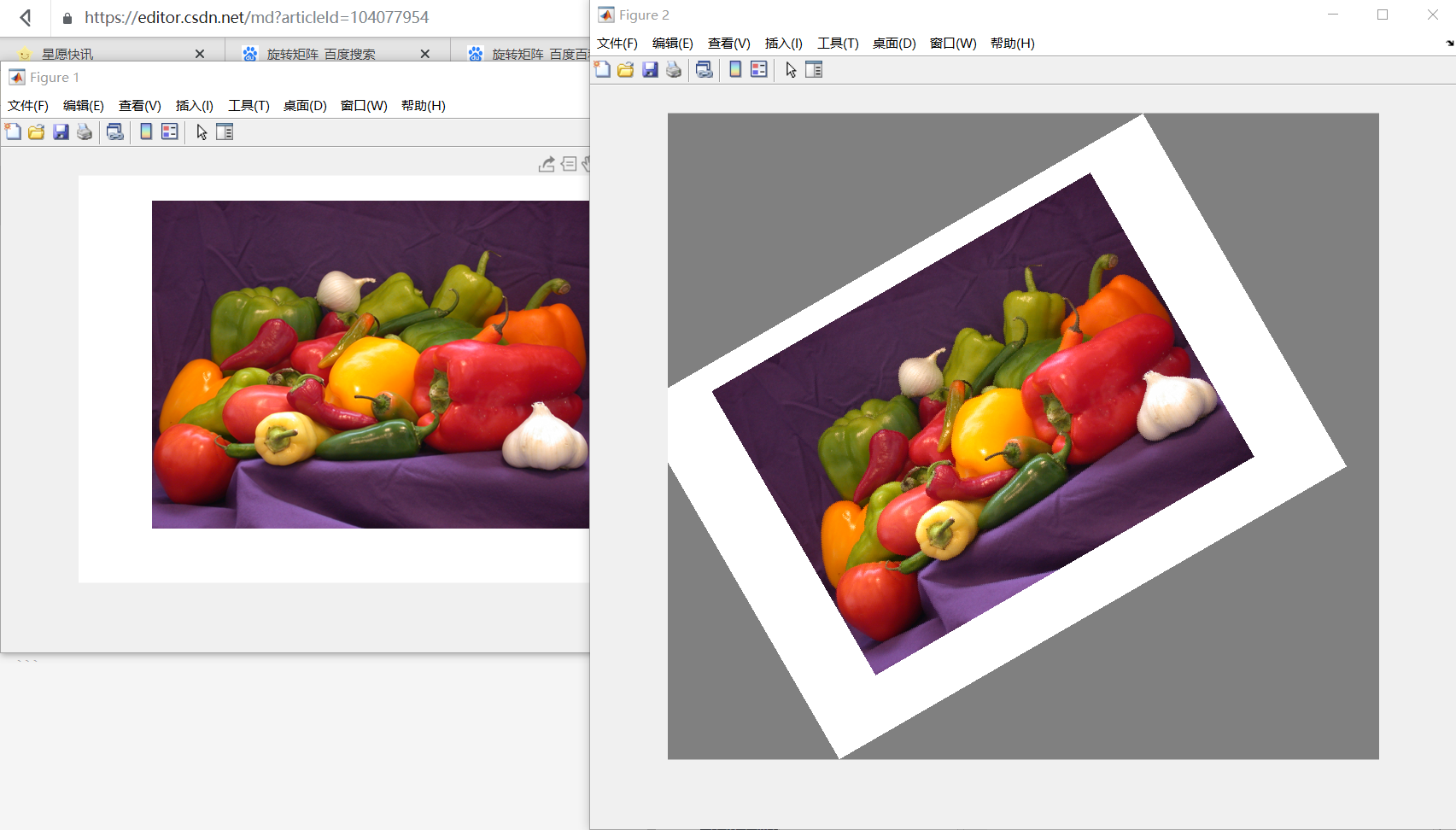可以看出这次的显示效果好得多了已经不存在像素点缺失的问题了实际上如果再进行双线性差值，图像的旋转效果会更好，我准备在下一篇和双线性差值在图像旋转中的应用一起展示，在这就不展示了。

展开全文计算机视觉 matlab
• matlab 的imrotate 函数对二值图像旋转时，不能实现功能，自己写了个旋转程序。旋转的基本原理如下：对原图中的点A(X0，Y0),旋转到(X1，Y1), 旋转角度为alpha，则 X1=X0*cos(alpha) +Y0*sin(alpha); Y1=-X0*sin...

第一次写博客，呵呵，先练习练习。

用matlab 的imrotate 函数对二值图像做旋转时，不能实现功能，自己写了个旋转程序。旋转的基本原理如下：对原图中的点A(X0，Y0),旋转到(X1，Y1),  旋转角度为alpha，则 X1=X0*cos(alpha) +Y0*sin(alpha); Y1=-X0*sin(alpha) +Y0*cos(alpha);

操作思路：

(1)在宽、高分别为Src_h,Src_w原图中找一点A(col,row), col ,row 为像素所在的列数和行数。

(2)建立坐标系，以图像中心为坐标原点。则点A(col，row)的坐标为 x=col -Src_w /2 , y=0.5* Src_h-row ;

(3) 在原来图像上找四个顶点并求出坐标值，按照旋转公式计算旋转后的图像大小，并求出旋转后图像对应原图的像素值，用邻近插值法或者双线性插值法解决浮点问题。

%对图像顺时针旋转alpha ，alpha为弧度制，旋转角度范围 0-360

function Dest_img=rot2 (Src_img,alpha)

[Src_h,Src_w]=size(Src_img);

x1=-0.5*Src_w; y1= 0.5*Src_h;

x2=0.5*Src_w;  y2=0.5*Src_h;

x3=0.5*Src_w;  y3=-0.5*Src_h;

x4=-0.5*Src_w; y4=-0.5*Src_h;

%%计算新图像的四个角的坐标以图像中心为坐标原点

Destx1=cos(alpha)*x1+sin(alpha)*y1;

Desty1=-sin(alpha)*x1+cos(alpha)*y1;

Destx2=cos(alpha)*x2+sin(alpha)*y2;

Desty2=-sin(alpha)*x2+cos(alpha)*y2;

Destx3=cos(alpha)*x3+sin(alpha)*y3;

Desty3=-sin(alpha)*x3+cos(alpha)*y3;

Destx4=cos(alpha)*x4+sin(alpha)*y4;

Desty4=-sin(alpha)*x4+cos(alpha)*y4;

%的到旋转后图像的宽度和高度

Dest_w=max(abs(Destx1-Destx3),abs(Destx2-Destx4));

Dest_h=max(abs(Desty1-Desty3),abs(Desty2-Desty4));

Dest_img=zeros(Dest_h,Dest_w);

for i=1:Dest_h

for j=1:Dest_w

%转换到旋转前对应的坐标

% (i,j) 点对应的坐标为 dest_x=j-0.5*Dest_w dest_y=0.5*Dest_h-i

dest_x=j-0.5*Dest_w;

dest_y=0.5*Dest_h-i;

%获得对应的旋转前的坐标

src_x=dest_x * cos(alpha) -dest_y *sin(alpha);

src_y=dest_x * sin(alpha) +dest_y *cos(alpha);

if  (src_x>-0.5*Src_w+1) && (src_x<0.5*Src_w-1) && (src_y >-0.5*Src_h+1 )&& (src_y <0.5*Src_h-1)

%将旋转前的 坐标对应到图像的行和列 ,四舍五入，相当于最邻近插值

row=round(0.5*Src_h-src_y);

col=round(0.5*Src_w+src_x);

Dest_img(i,j) = Src_img(row,col);

end

end

end

效果如下：原图灰度化后旋转45度

参考文献

展开全文• 【数字图像处理】MATLAB实现图像旋转

万次阅读 多人点赞 2018-11-17 22:33:28
图像旋转原理 图像旋转的本质利用的是向量的旋转。 矩阵乘法的实质是进行线性变换，因此对一个向量进行旋转操作也可以通过矩阵和向量相乘的方式进行。 【ps：线性代数的本质这个视频很直观地解释了各种线性代数...数字图像处理 图像旋转
• matlab实现图像旋转

万次阅读 多人点赞 2016-10-07 14:30:30
要求 图像是彩色的；旋转后，原图像的四个角点应在新图像的四条...原理部分仅仅是代码中所体现的，具体推导过程参看百度文库 图像旋转 下图显示新图像与原图像的长宽关系，注意如果旋转角度是120度，则需要取...数字图像 matlab 图像旋转
• 文章目录四、matlb的imrotate函数一、图像旋转原理二、使用matlab实现1、思路2、实现代码3、优化1、思路2、代码实现3、使用双线性插值4、matlab自带的imrotate函数 四、matlb的imrotate函数 一、图像旋转原理  ...matlab 图像处理
• 本文采用基本原理图像进行旋转 使用MATLAB语言， 基本推导过程可参考https://blog.csdn.net/qq_42655135/article/details/88601342 一、程序 clear;close all;clc; gray=rgb2gray(imread('test1.jpg'));%转化...
• matlab imrotate图像旋转

千次阅读 2017-10-23 10:30:07
B = imrotate(A,angle) 将图像A（图像的数据矩阵，既可以是灰度图像，也可以是RGB图像）绕图像的中心点旋转angle度， 正数表示逆时针旋转， 负数表示顺时针旋转。返回旋转后的图像矩阵。   B = imrotate(A,angle...
• 数字图像处理（MATLAB）实验一一、实验名称图像的采样与量化二、实验目的1.熟悉MATLAB软件的使用。2.掌握采样与量化的原理及数学运算。3.于MATLAB环境下编程实现对图片的不同程度的采样与量化。三、实验内容1.对于...
• 可用于双线性插值旋转等等，MATLAB有强大的图像处理工具箱，可调用imrotate函数
• MATLAB图像投影原理

千次阅读 2019-09-25 08:51:50
• =摘要=图像处理老师的课很...当然掩盖不了心中的好奇心----图像旋转是怎么实现的?于是经过一定的探索(主要是matlab的用法)...终于实现了这个"旋转":)本文就是关于这个"探索"过程的一点文字记录啦.=预览一下=matlab...
• 你在图像中有洞的原因是...为此，您只需要应用逆变换，在旋转矩阵的情况下只是矩阵的转置(只需更改每个sin上的符号并以另一种方式转换)。在imagerot中循环像素：imagerot=zeros(size(imagepad)); % midx and midy...
• 在这里你将能够获取所有的源代码以及测试图片，完全可以在你自己的Matlab上跑这些程序。 如果你是学生，请注意不要抄袭，课程设计作业的话，这种程序仅仅只能让你得到80%左右的成绩。 如果你是工作人士或者只是感...倾斜校正
• 本代码为MATLAB实现图像的几何变换，包含图像的水平镜像、垂直错切、旋转和加倍，内附有图片经过代码处理后的结果
• 利用matlab进行图像的一些操作处理 缩放和旋转功能的实现
• 本文介绍了图像旋转的基本原理MATLAB实现，在不借助MATLAB自带函数的情况下，自己书写了实现图像旋转步骤的几个函数，使用的插值方法为双线性插值。图像旋转 MATLAB 双线性插值
• 对于看旋转原理，这里可以去看一位大佬写的文章，写的很好，慢慢按着他的方法，就会懂旋转的原理，这里我就不多说了。（写的真的很好！）文章链接 自己写的代码： function [newimage]=rotat...MATLAB 数字图像处理 图像旋转
• 汝强【摘 要】在空间解析几何教学中，MATLAB不仅能将复杂的空间曲面方程精准、直观地用三维图形表现出来，还能以动画的形式将空间复杂曲面任意旋转，使学生能直观地、全方位地观察、理解空间曲面方程所表示的曲面形...
• 图像旋转matlab

2011-04-02 11:56:59
matlab图像旋转的原始代码，有助于学习图像变换的基本原理图像
• MATLAB旋转体的绘制使用mesh绘制旋转原理：绘制旋转体，在于确定旋转的曲线以及所要绕着旋转的轴。例如，将一个YoZ平面上的半圆：z^2+(y-r)^2=1(z>=0)绕z轴旋转而成的旋转体。示意图如下： 当绕z轴旋转alpha...
• Matlab图像平移、旋转、缩放、镜像

千次阅读 多人点赞 2019-09-27 15:46:21
今天学习了用Matlab实现对图像的基本操作。在Matlab中，图像是按照二维矩阵的形式表示的。所以对图像的操作就是对矩阵的操作。 对图像进行缩放、平移、旋转，都可以转化为矩阵的运算。关于变换矩阵的构造，请参考：...
• matlab双线性插值实现彩色图像的平移、旋转、缩放、镜像原理概述代码平移镜像缩放旋转结果原图平移（向X正方向、Y正方向50个距离单位）镜像（左右、上下）缩放（查看分辨率可知是否成功）旋转（45°） 原理概述 图像...matlab
• Matlab:图像平移算法的原理实现，缺陷分析及优化 文章目录1.怎么样确定平移之后新的图片的模板呢?2.图像中的坐标平移转换是如何实现的呢？在实现平移算法之前，我们首先来了解平移算法的背景知识：那么我们如何将...算法 opencv 计算机视觉
• Matlab图形界面图像旋转、平移和缩放旋转、平移和缩放Matlab图形界面图像名称：类：学号：目录问题说明2摘要3正文41、界面设计42、打开图片功能43、5实现图中所有角度的旋转4、图像的平移85、缩放(放大和缩小)图像......

matlab图像旋转原理matlab 订阅